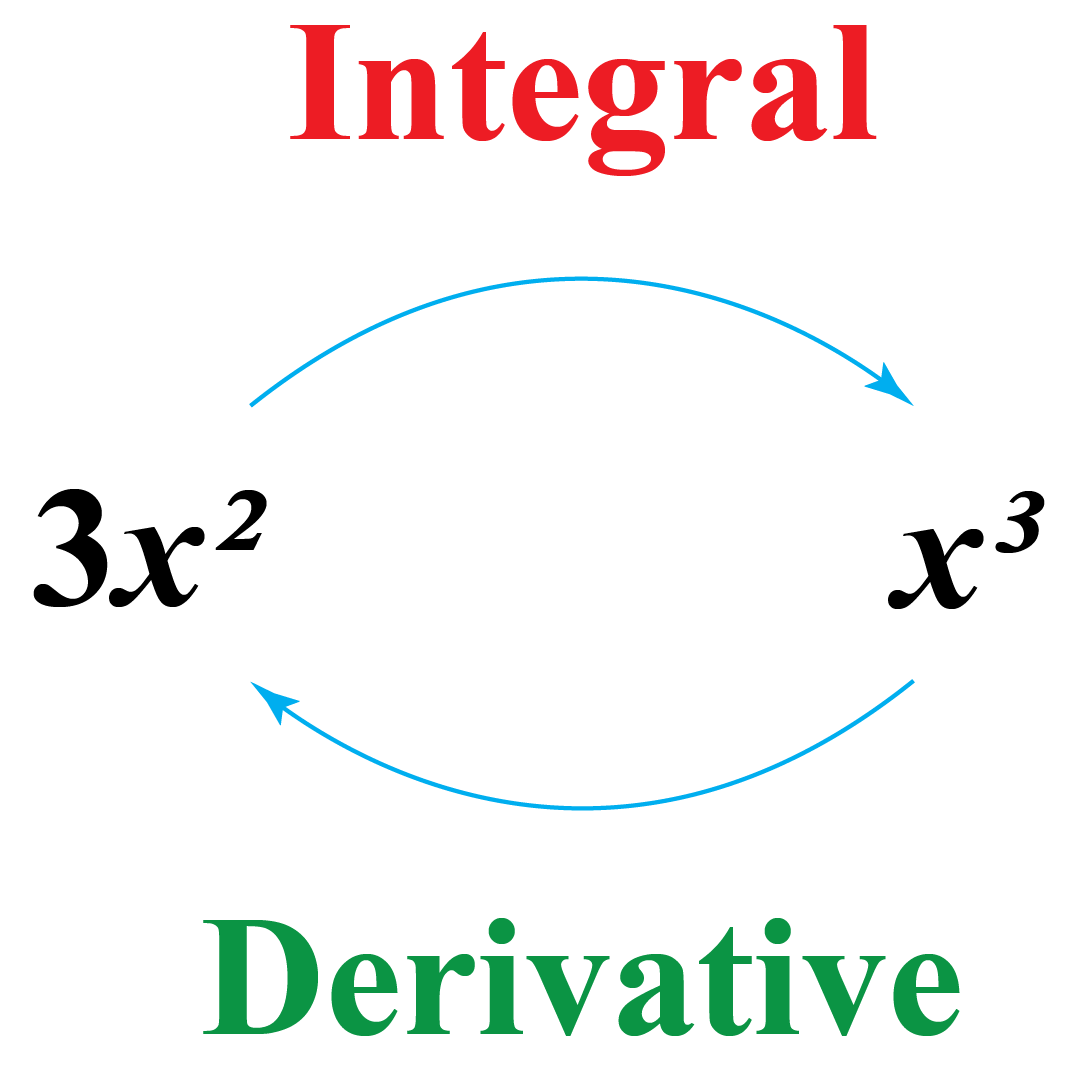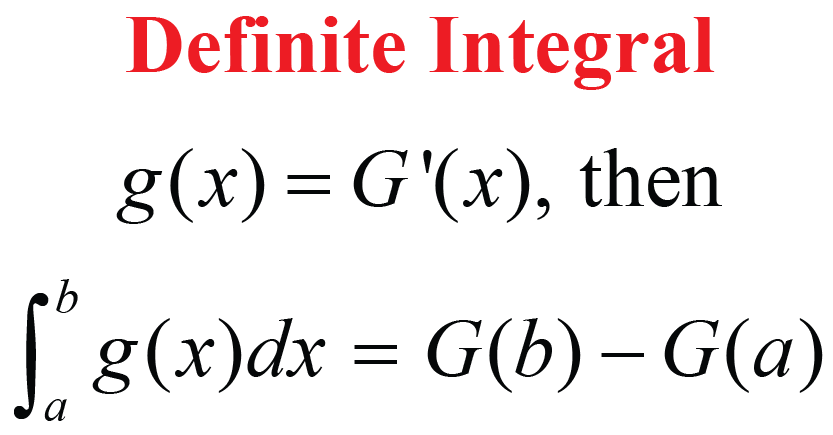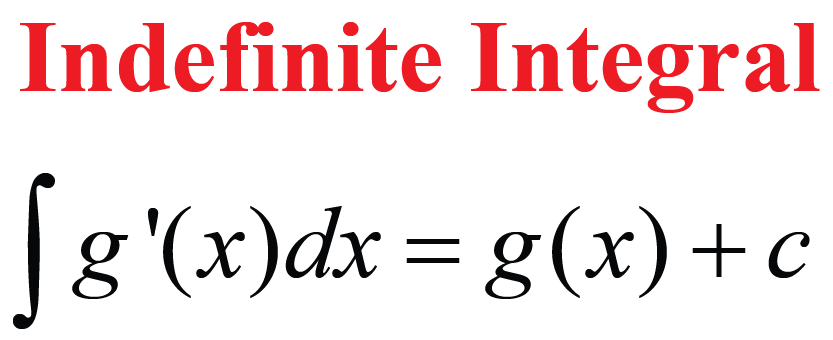# Integral

Integral

There is a very thin line of distinction between integration, integral, and derivative.

Let's try to understand it from the figure given here.In this topic, we will cover the basics of integration along with integral definition and types of integrals.

## Lesson Plan

 1 What Is the Meaning of Integral? 2 Important Notes on Integral 3 Solved Examples on Integral 4 Challenging Questions on Integral 5 Interactive Questions on Integral

## What Is the Meaning of Integral?

Given the derivative $$f’$$ of the function $$f$$, a question that arises is, "Can we determine the function$$f$$?"

Here, the function $$f$$ is called antiderivative or integral of $$f’$$.

The process of finding the antiderivative is called integration. On the other hand, the value of the function found by the process of integration is called an Integral

For example,

The derivative of $$f(x)=x^{3}$$ is $$f’(x)=3x^{2}$$;

And the antiderivative of $$g(x)=3x^{2}$$ is $$f(x)=x^{3}$$

Here, the integral of $$g(x)=3x^{2}$$ is $$f(x)=x^{3}$$

## What Are the Different Types of Integral?

In general, there are two types of integrals.

### Definite Integrals

These are the integrals that have a pre-existing value of limits; thus making the final value of integral definite.### Indefinite Integrals

These are the integrals that do not have a pre-existing value of limits; thus making the final value of integral indefinite.Here, $$c$$ is the integration constant.

## What Are the Integration Formulas?

We can remember the formulas of derivatives of some important functions.

Here are the corresponding integrals of these functions.

Derivatives Integrals
$$\dfrac{d}{dx}\left(\dfrac{x^{n+1}}{n+1}\right)=x^{n}$$ $$\int x^{n} dx=\dfrac{x^{n+1}}{n+1}+C$$, where $$n \neq -1$$
$$\dfrac{d}{dx}\left(x\right)=1$$ $$\int dx=x+C$$
$$\dfrac{d}{dx}\left(\sin{x}\right)=\cos{x}$$ $$\int \cos{x}dx=\sin{x}+C$$
$$\dfrac{d}{dx}\left(-\cos{x}\right)=\sin{x}$$ $$\int \sin{x}dx=-\cos{x}+C$$
$$\dfrac{d}{dx}\left(\tan{x}\right)=\sec^{2}{x}$$ $$\int \sec^{2}{x}dx=\tan{x}+C$$
$$\dfrac{d}{dx}\left(-\cot{x}\right)=\csc^{2}{x}$$ $$\int \csc^{2}{x}dx=-\cot{x}+C$$
$$\dfrac{d}{dx}\left(\sec{x}\right)=\sec{x}\tan{x}$$ $$\int \sec{x} \tan{x}dx=\sec{x}+C$$
$$\dfrac{d}{dx}\left(-\csc{x}\right)=\csc{x}\cot{x}$$ $$\int \csc{x} \cot{x}dx=-\csc{x}+C$$
$$\dfrac{d}{dx}\left(\sin^{-1}{x}\right)=\dfrac{1}{\sqrt{1-x^{2}}}$$ $$\int\left(\dfrac{1}{\sqrt{1-x^{2}}}\right)dx=\sin^{-1}{x}+C$$
$$\dfrac{d}{dx}\left(\cos^{-1}{x}\right)=-\dfrac{1}{\sqrt{1-x^{2}}}$$ $$\int\left(-\dfrac{1}{\sqrt{1-x^{2}}}\right)dx=\cos^{-1}{x}+C$$
$$\dfrac{d}{dx}\left(\tan^{-1}{x}\right)=\dfrac{1}{{1+x^{2}}}$$ $$\int\left(\dfrac{1}{{1+x^{2}}}\right)dx=\tan^{-1}{x}+C$$
$$\dfrac{d}{dx}\left(\cot^{-1}{x}\right)=-\dfrac{1}{{1+x^{2}}}$$ $$\int\left(-\dfrac{1}{{1+x^{2}}}\right)dx=\cot^{-1}{x}+C$$
$$\dfrac{d}{dx}\left(\sec^{-1}{x}\right)=\dfrac{1}{x\sqrt{x^{2}-1}}$$ $$\int\left(\dfrac{1}{x\sqrt{x^{2}-1}}\right)dx=\sec^{-1}{x}+C$$
$$\dfrac{d}{dx}\left(\csc^{-1}{x}\right)=-\dfrac{1}{x\sqrt{x^{2}-1}}$$ $$\int\left(-\dfrac{1}{x\sqrt{x^{2}-1}}\right)dx=\csc^{-1}{x}+C$$
$$\dfrac{d}{dx}\left(e^{x}\right)=e^{x}$$ $$\int e^{x}dx=e^{x}+C$$
$$\dfrac{d}{dx}\left(\ln{|x|}\right)=\dfrac{1}{x}$$ $$\int \dfrac{dx}{x}=\ln{|x|}+C$$
$$\dfrac{d}{dx}\left(\dfrac{a^{x}}{\ln{a}}\right)=a^{x}$$ $$\int a^{x} dx=\dfrac{a^{x}}{\ln{a}}+C$$

Try out different integral formulas in the given integral calculator. You can enter different values of random functions, and you will get their integrals here:

More Important Topics
Numbers
Algebra
Geometry
Measurement
Money
Data
Trigonometry
Calculus